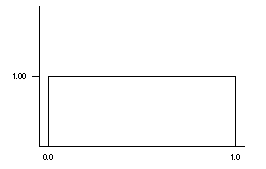### CFA Practice Question

There are 985 practice questions for this topic.

### CFA Practice Question

Let the random variable X be a random number with the uniform density curve given below.P(0.7 < X < 1.1) has value ______.

A. 0.30
B. 0.40
C. 0.70